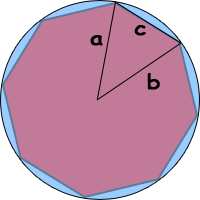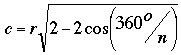SEARCH HOMEMath Central Quandaries & QueriesQuestion from lindsay, a student: how do find the perimeter of a regular octagon inscribed in a circle with a radius of 5 unitsHi Lindsay.

Here's a method that solves this problem for any regular n-gon inscribed in a circle of radius r.A regular n-gon divides the circle into n pieces, so the central angle of the triangle I've drawn is a full circle divided by n: 360°/n.

The Law of Cosines applies to any triangle and relates the three side lengths and a single angle, just as we have here. In general it says this:

 The Law of Cosines: For any triangle with side lengths a, b and c, c2 = a2 + b2 - 2ab cos C where angle C is the angle opposite side length c.
This is the key to the answer.

In the case of our regular n-gon, we have a = b = r and C = 360°/n, so we simplify it to:

c2 = r2 + r2 - 2rr cos (360°/n)
c2 = 2r2 - 2r2 cos (360°/n)
c
2 = r2 (2 - 2 cos (360°/n))

which makes a final equation:If you wanted the perimeter of the n-gon, you would just multiply this by n of course.

For your question Lindsay, just put 5 units in for r and 8 in for n and you can calculate c.

Hope this helps,
Stephen La RocqueMath Central is supported by the University of Regina and The Pacific Institute for the Mathematical Sciences.